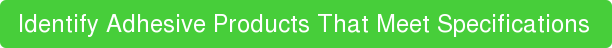ËModulus of elasticity, also know as "young's modulus," is a metric that represents a material's resistance to being deformed elastically. Throughout the site we use the terms "high modulus" and "low modulus" frequently to describe a cured polymer adhesive product. This post is dedicated to spelling out exactly what each of these terms mean.

Other polymer properties worth checking out:

# High Vs. Low, What Does It Mean?

For those of you just looking for the quick answer, here it is. Modulus of elasticity represents a material's resistance to being deformed, so low values mean low resistance and high values mean high resistance. In other words:

## High Modulus = Stiff

It's as simple as that.

# But How Is The Elastic Modulus Of A Material Specifically Defined?

For those of you looking for a little bit deeper of an explaination, we're going to have to work through some definitions.

• Strain is the amount a material is deformed (stretched) relative to its original dimensions
• Stress is the amount of force applied to the material and can be thought of as the material's resistance to strain (stretching).
• A Stress-Strain Curve is a graphical representation of the relationship between stress and strain for a particular material. Stress is plotted as a function of strain (strain along x-axis, stress along y-axis). The curve is basically how much the material will resist various amounts of stretching. If the curve has a high slope then it takes a lot of force to stretch the material a small amount (stiff). If the curve is has a low slope then it it takes very little force to stretch the material very far (flexible).
• The Elastic Deformation Region of a stress-strain curve is the region in which the material exhibits elastic behavior (if allowed the material will elastically return to its original dimensions).
• The Elastic Modulus of a material is the slope of the stress-strain curve in the elastic deformation region.

When we put all these definititons together this of course fits with our understanding above: the elastic modulus of a material represents a material's resistance to being deformed elastically.